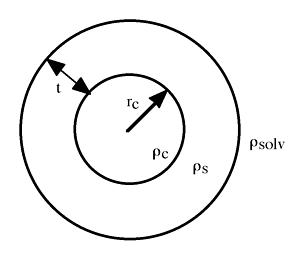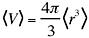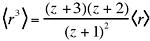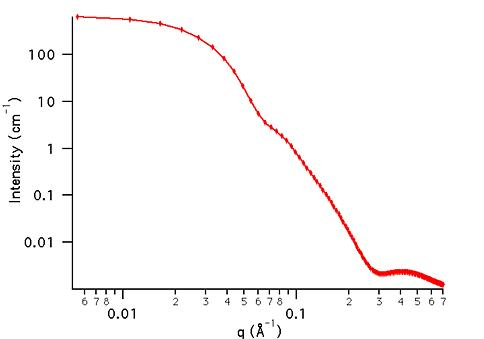MODEL

PolyCoreShellRatio

AUTHOR/MODIFICATION

Steve Kline 06 NOV 1998

Alan Munter 08 JULY 1999, converted to Java

APPROVED FOR DISTRIBUTION

DESCRIPTION

Calculates the form factor for a polydisperse spherical particle with a core-shell structure. In this model, the ratio R(core)/R(core+shell) is held constant. The form factor is normalized by the mean particle volume such that P(q) = scale*<f2>/Vol + bkg, where f is the single particle scattering amplitude and the average < > is over a Schulz distribution of radii.

Resolution smeared version is also provided.

VARIABLES

Input Variables (default values):

Parameter Variable Value
0Scale1.0
2Average Shell Thickness (Å)10.0
3Overall Polydispersity (0-1)0.2
4Core SLD (Å-2)1.0e-6
5Shell SLD (Å-2)2.0e-6
6Solvent SLD (Å-2)3.0e-6
7incoherent Background(cm-1)0.000

USAGE NOTES

The returned value is scaled to units of [cm-1], on absolute scale.Parameter (scale) is correlated with the SLD's that describe the particle. No more than one of these parameters can be free during model fitting.

Parameter (polydispersity) is constrained to keep it within its physical limits of (0,1). If a value larger than 1 is entered it is quietly changed to 1 for the calculation. Polydispersity, p = s/Rt, where s2 is the variance of the distribution and Rt is the total particle radius, Rt = Rc +t. For a more complete description of the Schulz distribution, see: J. Hayter in "Physics of Amphiphiles - Micelles, Vesicles and Microemulsions" V. DeGiorgio and M. Corti, Eds. (1983) p. 69.

The returned form factor is normalized by the average particle volume <V>:whereand here r denotes the total particle radius, Rc + t, and z is the width parameter of the Schulz distribution,.

If the scale factor Parameter is set equal to the particle volume fraction, phi, the returned value is the scattered intensity per unit volume, I(q) = phi*P(q). However, no interparticle interference effects are included in this calculation.

REFERENCE

J. B. Hayter in "Physics of Amphiphiles--Micelles, Vesicles, and Microemulsions" Eds. V. DeGiorgio; M. Corti, pp. 59-93,1983. Eqns: 32-37

TEST DATASET

This example dataset is produced by calculating the PolyCoreShellRatio using 128 data points, qmin = 0.001 Å-1, qmax = 0.7 Å-1 and the above default parameter values.